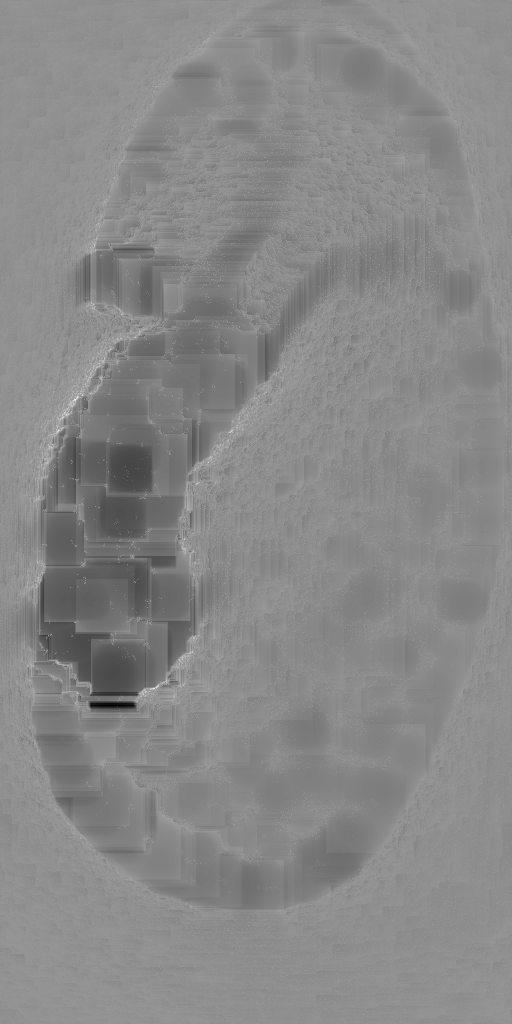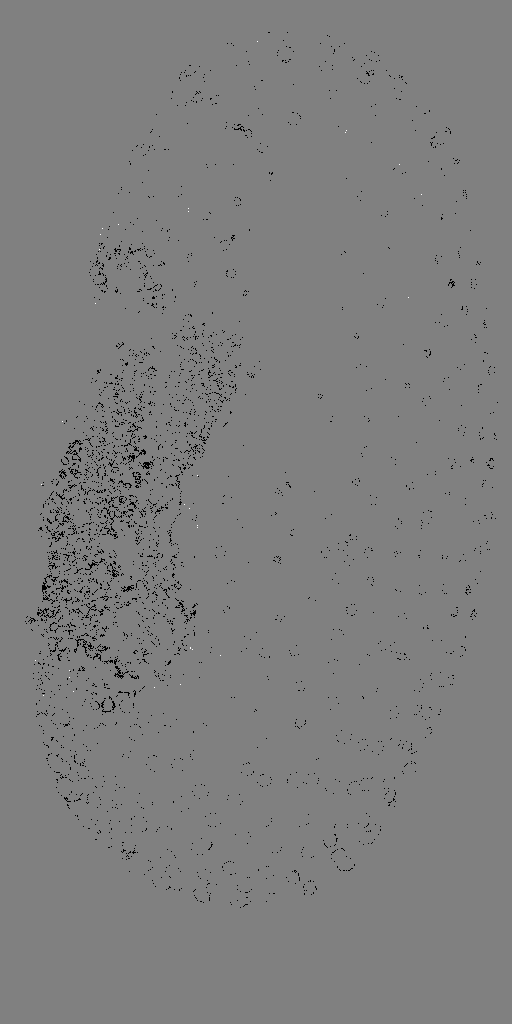# CLIJ2GPU accelerated image processing for everyone

CLIJ2 home

# Comparing Workflows: ImageJ vs CLIJ

Authors: Robert Haase, Daniela Vorkel, March 2020

Source

This macro shows how to compare an ImageJ based workflow and its translation using CLIJ.

Let’s start with ImageJ: we have a workflow to load an image and to process it with background subtraction and thresholding. Note the two lines using the `getTime();` command.

```run("Close All");

// get test data
open("https://github.com/clij/clij2-docs/raw/master/src/main/resources/Lund_MAX_001300.tif");
rename("input_imagej");

start_time_imagej = getTime();

// subtract background in the image
run("Duplicate...", "title=background_subtracted_imagej");
run("Subtract Background...", "rolling=25");

// threshold the image
run("Duplicate...", "title=thresholded_imagej");
setAutoThreshold("Default dark");
setOption("BlackBackground", true);

end_time_imagej = getTime();

```

Now, we run the same workflow with CLIJ methods:

```
// get test data
open("https://github.com/clij/clij2-docs/raw/master/src/main/resources/Lund_MAX_001300.tif");
input_clij = getTitle();

start_time_clij = getTime();

// init GPU
run("CLIJ2 Macro Extensions", "cl_device=RTX");
Ext.CLIJ2_clear();

// push data to GPU
Ext.CLIJ2_push(input_clij);

// subtract background in the image
background_subtracted_clij = "background_subtracted_clij";
Ext.CLIJ2_topHatBox(input_clij, background_subtracted_clij, 25, 25, 0);
Ext.CLIJ2_pull(background_subtracted_clij);

// threshold the image
thresholded_clij = "thresholded_clij";
Ext.CLIJ2_automaticThreshold(background_subtracted_clij, thresholded_clij, "Default");
Ext.CLIJ2_pullBinary(thresholded_clij);

end_time_clij = getTime();

```

The results look similar. There are differences, because the implementation of ImageJ background subtraction is close but not identical to the CLIJs topHatBox filter. Furthermore, CPUs and GPUs run computations a bit differently.

## Quantitative image comparison

You may have noticed that all intermediate images got new names. This allows us to compare them, now.

Let’s start with quantitative measurements on images and take the duration time for processing.

```// clean up and configure measurements
run("Set Measurements...", "area mean standard min redirect=None decimal=3");
run("Clear Results");

// measure the image using the ImageJ workflow
selectWindow("background_subtracted_imagej");
run("Measure");
selectWindow("thresholded_imagej");
run("Measure");

// measure the image using the CLIJ workflow
selectWindow("background_subtracted_clij");
run("Measure");
selectWindow("thresholded_clij");
run("Measure");

// Table.rename("Results", "Quantitative measurements");

```

From these measurements, we conclude that there are small differences between background-subtracted images, and apparently smaller differences between binary result images.

We can verify that by visualizing the differences. Note that we choose to save the subtracted images in 32-bit, because 8-bit images don’t support negative values.

## Visual differences between background-subtracted images

```imageCalculator("Subtract create 32-bit", "background_subtracted_imagej", background_subtracted_clij);

```## Visual differences between thresholded images

```imageCalculator("Subtract create 32-bit", "thresholded_imagej", thresholded_clij);

```The visualization confirms our assumption: The background-subtracted images are slightly different, while the binary result images are not.

## Comparing processing time

Also, let’s compare the different processing times:

```
print("ImageJ took " + (end_time_imagej - start_time_imagej) + "ms.");
print("CLIJ took " + (end_time_clij - start_time_clij) + "ms.");

```
```> ImageJ took 410ms.
> CLIJ took 93ms.
```

The shown numbers depend on the used GPU hardware. Therefore, it’s a good practice to document which GPU was used:

```
Ext.CLIJ2_getGPUProperties(gpu, memory, opencl_version);
print("GPU: " + gpu);
print("Memory in GB: " + (memory / 1024 / 1024 / 1024) );
print("OpenCL version: " + opencl_version);

```
```> GPU: GeForce RTX 2060 SUPER
> Memory in GB: 8
> OpenCL version: 1.2
```

Note: If you run this script a second time, the shown numbers may differ a bit, especially when CLIJ becomes faster, because the so called warmup period is over. During the first period, code gets compiled. This compilation takes time and thus, when done for a second time, the processing can be significantly faster. Furthermore, there are always fluctations in time measurements. Therefore, it is recommended to run such workflows many times in a loop, and doing statistics on its derived measurements.

Last but not least, clean up by closing all windows and empty GPU memory:

```
run("Close All");
Ext.CLIJ2_clear();
```